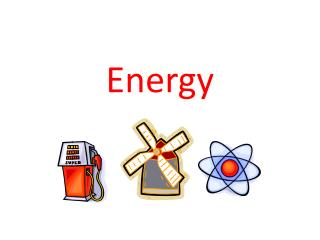# Energy - PowerPoint PPT PresentationDownload PresentationEnergy

EnergyDownload Presentation## Energy

- - - - - - - - - - - - - - - - - - - - - - - - - - - E N D - - - - - - - - - - - - - - - - - - - - - - - - - - -
##### Presentation Transcript

1. Energy

2. Energy Equations • Remember the Law of Conservation of Energy? • Well energy equations show how this energy is transformed e.g. Battery • Chemical potential energy → electrical energy • E.g Toaster • Electrical energy → Heat energy + light energy

3. Answer these energy equations • In your notebook complete these energy equations: • Flying Airplane • Light bulb • Moving Car • Computer Remember that energy is transformed (changed) Into different forms. It can be transferred (moved to) different objects.

4. Answers to energy equations • Flying Airplane Chemical potential energy → Kinetic+Heat+Sound • Light bulb Electrical energy → Heat+Light Energy • Moving car Chemical potential energy → Kinetic+Sound+Heat • Computer Electrical energy → Heat+Light+Soundenergy

5. Energy words! • The following words are words you need to know for this topic: Joules – unit of measurement for energy Watt – unit of measurement for power Kinetic energy – energy of moving objects Chemical energy – energy in food and fuels Electrical energy – most useful form of energy Friction – a force that opposes motion. Friction can cause energy to be lost as heat and sound.

6. Perpetual Motion • YouTube - Perpetual Motion machine • This is a machine that continues to move once set in motion – forever! Is this possible? Why/why not. • Take some time to think this out, after you have an idea share with your partner. • (Think, pair, share)

7. Are you cold? • What is hypothermia? • This is when our body temperature is lowered. Warm blooded animals require a constant body temperature for their bodies to carry out life processes. Humans have a body temperature of 37⁰C. If this is lowered by only 1 – 2 ⁰ hypothermia starts to set in. • Once this process sets in shivering sets in, this is the bodies way of trying to convert chemical energy to kinetic and heat energy to try to raise the body temperature.

8. Renewable/Non-renewable • Fossil fuels such as coal, oil and natural gas are non-renewable forms of energy. • http://www.umich.edu/~gs265/society/fossilfuels.htm • What are two reasons why fossil fuels are not a good source of energy? • Non-renewable • Create pollution